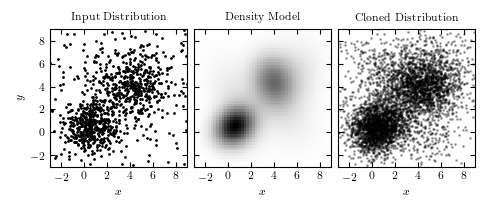# Cloning a Distribution with Gaussian Mixtures¶

Figure 6.10

Cloning a two-dimensional distribution. The left panel shows 1000 observed points. The center panel shows a ten-component Gaussian mixture model fit to the data (two components dominate over other eight). The third panel shows 5000 points drawn from the model in the second panel.```
```
```# Author: Jake VanderPlas
#   The figure produced by this code is published in the textbook
#   "Statistics, Data Mining, and Machine Learning in Astronomy" (2013)
#   To report a bug or issue, use the following forum:
import numpy as np
from matplotlib import pyplot as plt

from sklearn.mixture import GaussianMixture

#----------------------------------------------------------------------
# This function adjusts matplotlib settings for a uniform feel in the textbook.
# Note that with usetex=True, fonts are rendered with LaTeX.  This may
# result in an error if LaTeX is not installed on your system.  In that case,
# you can set usetex to False.
if "setup_text_plots" not in globals():
from astroML.plotting import setup_text_plots
setup_text_plots(fontsize=8, usetex=True)

#------------------------------------------------------------
# Create our data: two overlapping gaussian clumps,
# in a uniform background
np.random.seed(1)
X = np.concatenate([np.random.normal(0, 1, (200, 2)),
np.random.normal(1, 1, (200, 2)),
np.random.normal(4, 1.5, (400, 2)),
9 - 12 * np.random.random((200, 2))])

#------------------------------------------------------------
# Use a GaussianMixture to model the density and clone the points
gmm = GaussianMixture(5, 'full').fit(X)
X_new = gmm.sample(5000)

xmin = -3
xmax = 9
Xgrid = np.meshgrid(np.linspace(xmin, xmax, 50),
np.linspace(xmin, xmax, 50))
Xgrid = np.array(Xgrid).reshape(2, -1).T

dens = np.exp(gmm.score_samples(Xgrid)).reshape((50, 50))

#------------------------------------------------------------
# Plot the results
fig = plt.figure(figsize=(5, 2))
bottom=0.12, top=0.9)

# first plot the input
ax.plot(X[:, 0], X[:, 1], '.k', ms=2)

ax.set_title("Input Distribution")
ax.set_ylabel('\$y\$')

# next plot the gmm fit
ax.imshow(dens.T, origin='lower', extent=[xmin, xmax, xmin, xmax],
cmap=plt.cm.binary)

ax.set_title("Density Model")
ax.yaxis.set_major_formatter(plt.NullFormatter())

# next plot the cloned distribution